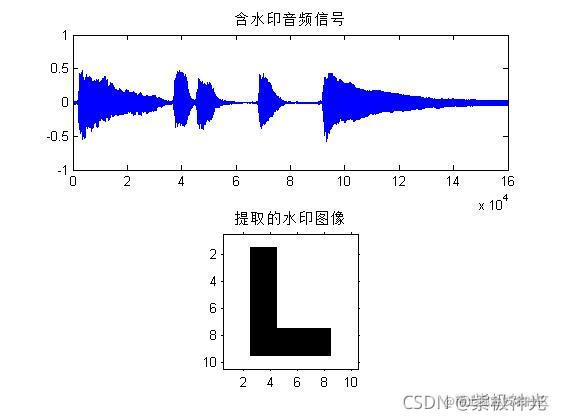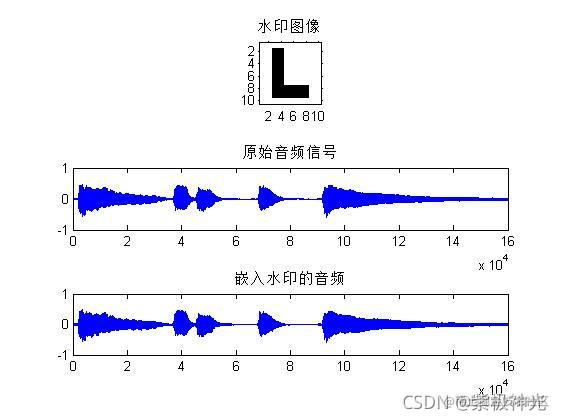# 【语音隐写】基于matlab DCT+DWT+SVD音频数字水印嵌入提取【含Matlab源码 1408期】

## 一、离散小波变换的音频信号数字水印技术简介

0 引言 近年来, 数字水印技术的作用越来越重要。数字水印技术是将一些标识信息直接嵌入数字载体当中, 或间接表示在信号载体中, 且不影响原载体的使用价值。通过隐藏在载体中的这些信息, 可以判断信息是否被篡改, 具有防伪溯源、保护信息安全、版权保护等作用。对于广播转播台站而言, 是广播音频的中转站, 在广播信号发送至千家万户之前务必保证信号的安全可靠, 但现在的大部分台站只是利用人耳的判断, 以及不同信源之间的比较, 具有较大的局限性。若利用数字水印的特性, 应用于广播节目中可以有效地防止信号插播, 可靠地保护信号安全, 保障广播的安全播出。

1 音频数字水印技术分类 根据数字水印在音频信号中的处理技术, 可将数字水印分为时域、变换域、压缩域数字水印。

1.1 时域数字水印 在时域数字水印技术中, 直接将水印信息嵌入至音频信号中, 通常会选择隐藏在信号不重要部位, 以保证其嵌入水印不影响原音频信号的监听效果。时域水印技术的实现较为容易且运算量小, 简单直接, 但是鲁棒性差, 容易被破解, 抵抗力较差。

1.2 变换域数字水印 在变换域数字水印中, 音频信号需经过时域至变换域的转换, 通常的变换域有离散余弦变换 (DCT, Discrete Cosine Transform) 、离散傅立叶变换 (DFT, Discrete Fourier transform) 、离散小波变换 (DWT, Discrete Wavelet Transform) 等。在变换域中嵌入水印信息, 通过反变换得到嵌入水印的音频时域信号。变换域水印技术较时域水印技术复杂, 但变换域嵌入的水印信息较时域而言, 不可见性更强, 隐蔽性更好, 鲁棒性更好。本文的研究主要基于DWT的音频信号的水印信息的嵌入与提取。

1.3 压缩域数字水印 在时域和变换域的水印技术, 都是直接将水印信号嵌入未压缩的音频格式中, 但是通常在音频信号的传输或存储中需要对音频信号进行压缩编码 (例如WMA、MP3等) , 因此压缩域数字水印也是水印技术也具有较大的实用价值。压缩域数字水印技术大致可分为三类: (1) 在非压缩域嵌入水印, 将音频信号与水印信息一起压缩; (2) 在压缩域中, 直接将水印信息嵌入压缩的音频信号中; (3) 将压缩后的信号进行解压缩, 然后嵌入水印信息, 最后将水印信息和解压后的音频信号一起压缩。总的来说, 压缩域水印技术的编解码系统过于复杂, 受压缩编码格式限制大, 压缩后的音频信号已经去除了冗余, 因此加入水印的难度大, 压缩域水印技术有待进一步研究。

2 基于DWT的音频水印算法 2.1 水印嵌入 本文研究的音频水印算法是基于离散小波变换 (DWT) , 音频信号通过DWT变换, 在变换域中嵌入水印信息, 再经过逆变换 (IDWT) 从而得到嵌入水印的音频信号。水印嵌入原理框图如图1所示。

2.2 水印提取 为了保证信息安全, 在发送端发送嵌入水印的音频信号, 而在接收端为了确定音频信息的准确性, 我们通常需要提取水印以确保来源的真实性, 因此水印的提取技术也尤为重要。在水印提取过程中, 需要原始音频信号与嵌入水印的音频信号同时进行DWT, 再将两者参数进行分析比较提取出水印信息。水印提取原理框图如图2所示。

## 二、部分源代码

``````%读取原始语音
A=audio(1:160000);
AL=length(A);
%绘制原始音频图：
subplot(312);plot(A);
title('原始音频信号');

%读入水印图片
I=im2bw(I);
subplot(311);imshow(I);
title('水印图像');
[m,n]=size(I);
%将图片降维
piexnum=1;
for i=1:m
for j=1:n
w(piexnum,1)=I(i,j);
piexnum=piexnum+1;
end
end
wl=size(w);

%对原音频进行2级小波分解：
[c,l]=wavedec(A,2,'haar');
%提取2级小波分解的低频（高能量）高频（低能量）：
ca2=appcoef(c,l,'haar',2);
cd2=detcoef(c,l,2);
cd1=detcoef(c,l,1);
ca2L=length(ca2);

%DCT变换
ca2DCT=dct(ca2);
%分段
k=wl(1);   %段数
DL=ca2L/k;  %ca2每段的长度
j=1;
delta=0.5;
%分段进行水印嵌入
for i=1:k
ca22=ca2DCT(j:j+DL-1);
Y=ca22(1:DL/4);        %提取前1/4系数
Y=reshape(Y,10,10);

[U,S,V]=svd(Y);        %SVD分解
S1=S(1,1);
S2=S(2,2);
D=floor(S1/(S2*delta)); %判别式
%根据D的奇偶性进行水印嵌入
if(mod(D,2)==0)
if (w(i)==1)
S(1,1)=S(2,2)*delta*(D+1);
else
S(1,1)=S(2,2)*delta*D;
end
else
if (w(i)==1)
S(1,1)=S(2,2)*delta*D;
else
S(1,1)=S(2,2)*delta*(D+1);
end
end
``````

## 三、运行结果## 四、matlab版本及参考文献

1 matlab版本 2014a

2 参考文献 韩纪庆,张磊,郑铁然.语音信号处理（第3版）[M].清华大学出版社，2019. 柳若边.深度学习:语音识别技术实践[M].清华大学出版社，2019.